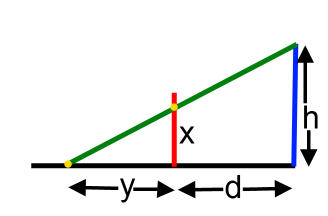#### You may also like### Fitting In

The largest square which fits into a circle is ABCD and EFGH is a square with G and H on the line CD and E and F on the circumference of the circle. Show that AB = 5EF. Similarly the largest equilateral triangle which fits into a circle is LMN and PQR is an equilateral triangle with P and Q on the line LM and R on the circumference of the circle. Show that LM = 3PQ### Look Before You Leap

Can you spot a cunning way to work out the missing length?### Triangle Midpoints

You are only given the three midpoints of the sides of a triangle. How can you construct the original triangle?

# It Depends on Your Point of View!

##### Age 14 to 16Challenge LevelImagine your eye is at the top of the blue line at a height of $h$. You want the image to appear to be standing straight up where the red line is, a distance $d$ horizontally from your eye.
For the yellow point to appear a height $x$ above the ground, it needs to be drawn a distance $y$ along the ground from the base of the red line.

If you knew $h$, $d$ and $x$, how could you work out $y$?